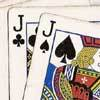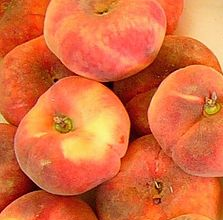#### You may also like### Do Unto Caesar

At the beginning of the night three poker players; Alan, Bernie and Craig had money in the ratios 7 : 6 : 5. At the end of the night the ratio was 6 : 5 : 4. One of them won $1 200. What were the assets of the players at the beginning of the evening?### Plutarch's Boxes According to Plutarch, the Greeks found all the rectangles with integer sides, whose areas are equal to their perimeters. Can you find them? What rectangular boxes, with integer sides, have their surface areas equal to their volumes?### 3388 Using some or all of the operations of addition, subtraction, multiplication and division and using the digits 3, 3, 8 and 8 each once and only once make an expression equal to 24. # Peaches Today, Peaches Tomorrow... ##### Age 11 to 14 Challenge Level: This problem is in three parts. If you are feeling confident about working with fractions, you might want to skip straight to part (ii) or part (iii).(i) A little monkey had 60 peaches. On the first day he decided to keep${\bf \frac{3}{4}}$of his peaches. He gave the rest away. Then he ate one. On the second day he decided to keep${\bf \frac{7}{11}}$of his peaches. He gave the rest away. Then he ate one. On the third day he decided to keep${\bf \frac{5}{9}}$of his peaches. He gave the rest away. Then he ate one. On the fourth day he decided to keep${\bf \frac{2}{7}}$of his peaches. He gave the rest away. Then he ate one. On the fifth day he decided to keep${\bf \frac{2}{3}}$of his peaches. He gave the rest away. Then he ate one. How many did he have left at the end? (ii) A little monkey had 75 peaches. Each day, he kept a fraction of his peaches, gave the rest away, and then ate one. These are the fractions he decided to keep: $$\frac{1}{2} \qquad \frac{1}{4} \qquad \frac{3}{4} \qquad \frac{3}{5} \qquad \frac{5}{6} \qquad \frac{11}{15}$$ In which order did he use the fractions so that he was left with just one peach at the end? (iii) Whenever the monkey has some peaches, he always keeps a fraction of them each day, gives the rest away, and then eats one. I wonder how long he could make his peaches last for... Here are his rules: • Each fraction must be in its simplest form and must be less than 1. • The denominator can never be the same as the number of peaches left. For example, if there were 45 peaches left, he could not choose to keep$\frac{44}{45}\$ of them.
Can you start with fewer than 100 peaches and choose fractions so that there is at least one peach left after a week?

Starting with fewer than 100, what is the longest you can make the peaches last?